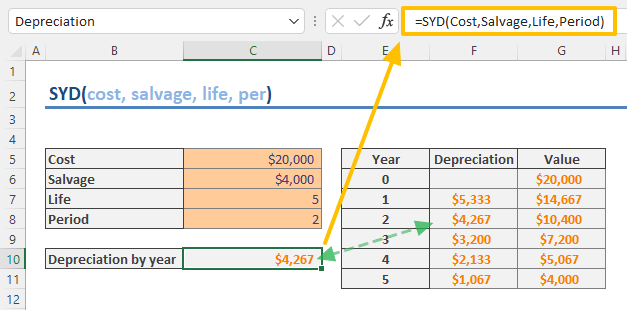The SYD function is a Finance function that calculates the depreciation of an asset on the sum-of-years' digits basis for a specified period. In this guide, we’re going to show you how to use the SYD function and go over some tips and error handling methods.

• All versions

## SYD Function Syntax

SYD(cost, salvage, life, per)

## Arguments

 cost The initial cost of the asset. salvage The value after the asset has been fully depreciated, salvage value. life The number of periods over which asset is depreciated, useful life of the asset. per Specific period to calculation depreciation for.

## SYD Function Example

Because all the arguments are required, the usage of the SYD function is straightforward. For example, for an asset with an initial cost of \$20,000, a useful life of 5 years, and a salvage value of \$4,000, the formula for the 2nd year should be as follows:

=SYD(20000,4000,5,2)

This formula returns the depreciation value for the specified period. The number of the period should match with the period you want to calculate the depreciation. If the life value is in years, the per value should also be a year.## Remarks

The sum-of-years' digits method can be calculated manually by the following formula:## Excel Depreciation Functions

 Straight line SLN Declining balance DB Double-declining balance DDB Variable-declining balance VDB French declining balance AMORDEGRC French straight line AMORLINC